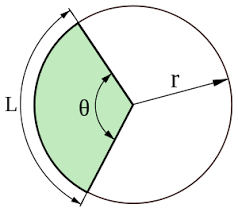# How to Calculate and Solve for the Angle, Radius and Length of an Arc of a Circle | The Calculator EncyclopediaThe image above represents the length of an arc of a circle.

To compute the length of an arc of a circle, two essential parameters are needed and this parameters are radius of the circle (r) and angle of the circle (α).

The formula for calculating the length of an arc of a circle:

L = απr / 180

Where;
L = Length of an arc of the circle
α = Angle of the circle
r = radius of the circle

Let’s solve an example:
Find the length of an arc of a circle when the angle of the circle is 90° and the radius of the circle is 20 cm.

This implies that;
α = Angle of the circle = 90°
r = Radius of the circle = 20 cm

L = απr / 180
L = 90 x 3.142 x 20 / 180
L = 5655.6 / 180
L = 31.42

Therefore, the length of an arc of the circle is 31.42 cm.

Calculating the Angle of a Circle using the Radius of the Circle and Length of an Arc of the Circle.

α = 180L / πr

Where;
L = Length of an arc of the circle
r = Radius of the circle

Let’s solve an example;
Find the angle of the circle when the length of an arc of the circle is 60° and a radius of 140 cm.

This implies that;
L = Length of an arc of the circle = 60°
r = Radius of the circle = 140 cm

α = 180L / πr
α = 180 x 60 / 3.142 x 140
α = 10800 / 439.88
a = 24.55

Therefore, the angle of the circle is 24.55°.

Calculating the Radius of a Circle using the Length of an Arc of the Circle and the Angle of the Circle.

r = 180L / πα

Where;
L = Length of an arc of the circle
α = Angle of the circle

Let’s solve an example;
Given that the angle of the circle is 100° with length of an arc of the circle 70 cm. Find the radius of the circle?

This implies that;
α = Angle of the circle = 100°
L = Length of an arc of the circle = 70 cm

r = 180L / πα
r = 180 x 70 / 3.142 x 70
r = 12600 / 219.94
r = 57.29

Therefore, the radius of the circle is 57.29 cm.

Nickzom Calculator – The Calculator Encyclopedia is capable of calculating the length of an arc of a circle.

To get the answer and workings of the length of an arc of a circle using the Nickzom Calculator – The Calculator Encyclopedia. First, you need to obtain the app.

You can get this app via any of these means:

To get access to the professional version via web, you need to register and subscribe for NGN 1,500 per annum to have utter access to all functionalities.
You can also try the demo version via https://www.nickzom.org/calculator

Once, you have obtained the calculator encyclopedia app, proceed to the Calculator Map, then click on Mensuration under the Mathematics sectionNow, click on Length of an Arc of a Circle under MensurationThe screenshot below displays the page or activity to enter your values, to get the answer for the length of an arc of a circle according to the respective parameters which are the radius of the circle (r) and angle of the circle (α).Now, enter the value appropriately and accordingly for the parameters as required by the example above where the radius of the circle is 20 cm and angle of the circle is 90°.Finally, Click on CalculateAs you can see from the screenshot above, Nickzom Calculator – The Calculator Encyclopedia solves for the length of an arc of a circle and presents the formula, workings and steps too.